Introduction

"I have 2000 features and I need to get to 50 features and keep the model as good or better" was how this all started. This is familiar to anyone in institutional data science where you need to carefully vet every model and look at each feature to make sure it is fine from the standpoint of regulation. What is valuable about this story is that it is based on a real production model that was developed by Anna Arakelyan at MassMutual and the Rainbow method suggested by Dmytro Karabash. We will get detailed results at the end but here's a preview for anyone who wants to take a peek:

Figure 1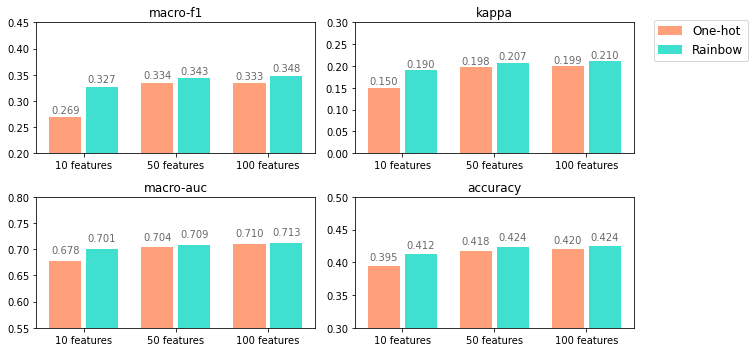The rainbow method outperforms the One-hot easily. The rainbow method even shows better results for 50 features than One-hot does for 100. Also note that when dropping from 50 to 10 features, the reduction in macro-f1 if you use One-hot is 6 times that of the rainbow method (3 times for kappa and accuracy, 2 times for macro-auc).

Both models keep improving as the number of features goes up. Though the improvement is substantially faster and larger for the rainbow method.

In this series of articles, we will introduce the Rainbow encoding method and will discuss its advantages over the conventional One-hot.
Part I below explains how the method works, shows that it preserves full data signal, and provides examples of its use for various kinds of categorical variables. Part II explores its empirical application to a real business data science problem - Mindset Segmentation model at MassMutual using the Acxiom database. Part III provides the mathematical foundation for the method and shows that it reduces model complexity and helps avoid overfitting.

Background

Data encoding is a crucial part of any data science pipeline. No matter which machine learning algorithm is used - there is no avoiding of data cleaning and encoding. Especially with dirty and complex real data sets, ensuring the most appropriate and efficient way of feature encoding and engineering is a nontrivial problem. While encoding quantitative and binary columns is usually a straightforward task, the encoding of categorical variables deserves a deeper look.

One of the most popular encoding methods for categorical variables has been the One-hot procedure. It generates an indicator variable for every category and, thus, creates a set of K new features, where K is the number of categories. One significant implication of this method is the dimensionality increase. Depending on the magnitude of K, it can have various undesirable technical consequences: substantial raise of computational complexity, loss of degrees of freedom (N-K, where N is the number of samples), multicollinearity, and, ultimately, an overfitted model. Non-technical consequences include unnecessary complications of the model that should be communicated to final users and that contradict the law of parsimony aka Occam's razor.

Method

We call it rainbow from a very simple analogy: if you have a categorical column with value names "Red", "Orange", "Yellow", "Green", "Blue", "Indigo" and "Violet", in other words - colors of the rainbow, instead of One-hot encoding, you can simply create one feature with encoding:

0 = "Red"
1 = "Orange"
2 = "Yellow"
3 = "Green"
4 = "Blue"
5 = "Indigo"
6 = "Violet"

This nicely replaces seven One-hot features with one rainbow.

Most of the nominal variables, at the first glance, seem like they cannot be converted to a quantitative scale. This is where we suggest finding a rainbow.
The seemingly unordered categories acquire a perfect order if we find a scale, where each category fits into its unique place. With the color - the natural scale is Hue. But it is not the only one. We can think of, say, brightness, saturation, color temperature, etc. We invite you to experiment with a few different rainbows that might capture different nuances of the categorical quality.

You can actually make and use two or more rainbows out of one categorical variable depending on the number of categories K and the context.

We don't recommend using more than $\log_2 {K}$ rainbows because we do not want to surpass the number of encodings in a binary One-hot.

Why is rainbow better than any other choice? It is very simple and intuitively sensible. In many cases it is not even that important which rainbow you choose (and by that we mean the color order), it would still be better than using One-hot. The more natural orders are just likely to be slightly better than others and easier to interpret.

Hence our motto:"When nature gives you a rainbow, take it..."

Important note: this method is highly efficient in conjunction with the models that rely on variables as ranks rather than exact values. For example, decision trees, random forest, gradient boosting - these algorithms will output the same result if, say, the variable Number of Children is coded as

0 = "0 Children"
1 = "1 Child"
2 = "2 Children"
3 = "3 Children"
4 = "4 or more Children"

or as

1 = "0 Children"
2 = "1 Child"
3 = "2 Children"
4 = "3 Children"
5 = "4 or more Children"

or even as

-100 = "0 Children"
-85 = "1 Child"
0 = "2 Children"
10 = "3 Children"
44 = "4 or more Children"

as long as the correct order of the categories is preserved. The values themselves don't carry the quantitative function in these algorithms. It is the rank of the variable that matters, and a tree-based algorithm will use its magic to make the most appropriate splits to introduce new tree nodes.

The application of our method for other algorithms such as Linear Regression, Logistic Regression is out of the scope of this article. We expect that such a way of feature engineering would be still beneficial, but that is a subject of a different investigation.

Note that by using a rainbow rather than One-hot we are not losing any of the data signal. Let's explore that question below.

Rainbow keeps the full signal

Let's zoom in for a minute on a classical rainbow example with just 4 values:

Color

0 = "Red"
1 = "Yellow"
2 = "Green"
3 = "Blue"

In the case of One-hot, we would create 4 features:

Color_Red = 1 if Color = 0 and 0 otherwise,
Color_Yellow = 1 if Color = 1 and 0 otherwise,
Color_Green = 1 if Color = 2 and 0 otherwise,
Color_Blue = 1 if Color = 3 and 0 otherwise.

In the case of Rainbow, we would just use the Color itself.

Let's compare the possible models made using these two methods: 4 features vs 1 feature. Let's for simplicity build a single decision tree. Let's look at a few scenarios of the data generating process.

Scenario 1

Assume all the categories are wildly different, and each one introduces a substantial gain to the model. That means each One-hot feature is indeed critical - the model should separate between all 4 groups created by One-hot.

In that case, an algorithm such as xgboost will just make the splits between all the values which is perfectly equivalent to One-hot. So the same exact result is achieved with just one feature instead of four.

Figure 2

One can clearly see that this example is easily generalized to One-hot with any number of categories. Also, note that for such data generating process the order of the categories in Rainbow does not even matter as splits will be made between all categories. In practice, (K-1) splits will be sufficient for both methods to separate between K categories.

Figure 3

The main takeaway is that not a bit of data signal is lost if one switches from One-hot to Rainbow. In addition to this, depending on the number of categories, a substantial dimensionality reduction happens which is beneficial for multiple reasons.

Scenario 2

If the chosen rainbow (Figure 3) is not the best because, for example, the data generating process separates between the group of {Red, Green} and {Yellow, Blue}, then once again - the algorithm will make all the necessary splits.

Figure 4

Here we show that depending on the order of binary features picked by One-hot there can be different trees built. In the case of Rainbow, though - once again we demonstrate that no information is lost and a (K-1) splits tree is built even in this least favorable scenario.

Scenario 3

Finally, if the data generating process is actually in agreement with the Rainbow order, then the Rainbow method would be superior to One-hot. Not only will it not lose any data signal, but it will also substantially reduce complexity, decrease dimensionality, and help avoid overfitting.

Suppose, the true model pattern only separates between {Red, Yellow} and {Green, Blue}. In that case, Rainbow has a clear advantage as it exploits these groupings while One-hot does not.

Figure 5

In this introductory article, we briefly show that by substituting One-hot with Rainbow, we save dimensionality without any informational loss. In Part III though we dive deeper into the mathematical foundation of the Rainbow method and prove that the Rainbow method outperforms One-hot in the worst, average, and best scenarios.

In short, if the rainbow order is even somewhat meaningful, the algorithm would capture the similarity in the adjacent values by making a smart decision about the number and location of splits. Thus, we will receive a simpler and better model.

Examples of Categorical Variables

The application of our method depends highly on the level of measurement of the treated variables. While quantitative variables have a ratio scale, i.e. they have a meaningful 0, ordered values, and equal distances between values; categorical variables usually have either interval, or ordinal, or nominal scales. Let us illustrate our method for each of these types of categorical variables.

Interval variables have ordered values, equal distances between values, but the values themselves are not necessarily meaningful, for example, 0 does not indicate an absence of some quality. The common examples of interval variables are Likert scales:

How likely is the person to buy a smartphone mobile phone?

1 = "Very Unlikely"
2 = "Somewhat Unlikely"
3 = "Neither Likely Nor Unlikely"
4 = "Somewhat Likely"
5 = "Very Likely"

Straightforwardly, if we simply use the raw values 1 through 5, that will be the best and most natural rainbow. The algorithm such as xgboost will make the appropriate splits instead of being forced to use the splits predetermined by One-hot, which in many cases is simply an overfit.

Ordinal variables have ordered meaningless values, and the distances between values are also not equal or not even explainable. An example:

What is the highest level of Education completed by the person?

1 = "No High School"
2 = "High School"
3 = "Associate Degree"
4 = "Bachelor's Degree"
5 = "Master's Degree"
6 = "Doctoral Degree"

Similar to interval variables, raw ordinal numeric codes can be used in the model without introducing any issues, as long as the order is correct. In some cases, a variable is intrinsically ordinal, but the given numeric codes don't follow the correct order - in these situations, we could simply reorder categories and then use this updated variable as a quantitative feature.

The use of a natural rainbow for interval and ordinal variables is likely not the cause for concern because it is clearly a perfect alternative to One-hot for tree-based algorithms. The more complicated and non-obvious question is how to treat nominal variables.

Nominal variables have no obvious order between categories and are almost always encoded with the One-hot method. This is where finding a rainbow becomes valuable. It will turn something, that seems completely nonquantitative, into a quantitative scale. In our classical example, a nominal variable Color

A = "Red"
B = "Blue"
C = "Green"
D = "Yellow"

can be replaced by the newly engineered rainbow feature

1 = "Blue"
2 = "Green"
3 = "Yellow"
4 = "Red"

Lets us show a few other examples of creating rainbows.

Vehicle Type

C = "Compact Car"
F = "Full-size Car"
L = "Luxury Car"
M = "Mid-Size Car"
P = "Pickup Truck"
S = "Sports Car"
U = "SUV"
V = "Van"

For this variable, we can think of dozens of characteristics to make a rainbow - vehicle size, capacity, price category, average speed, fuel economy, costs of ownership, motor features, etc. Which one (or more than one) to pick? The choice depends on the context of the model and what are you trying to predict. The preference should be given to the ones that provide more explanatory power and/or make sense from the interpretation standpoint.

Consider another variable:

Marital Status

A = "Married"
B = "Single"
C = "Inferred Married"
D = "Inferred Single"

If we think about Single and Married as of the two ends of the spectrum, then Inferred Single could be between the two ends, closer to Single, while Inferred Married would be between the two ends, closer to Married. That would make sense because Inferred holds a certain degree of uncertainty. Thus, the following order would be reasonable:

1 = "Single"
2 = "Inferred Single"
3 = "Inferred Married"
4 = "Married"

In case there are any missing values here, the Unknown category fits exactly in the middle between Single and Married as there is no reason to prefer one end over the other. So the modified scale could look like this:

1 = "Single"
2 = "Inferred Single"
3 = "Unknown"
3 = "Inferred Married"
4 = "Married"

Another example:

Occupation

1 = "Professional/Technical"
3 = "Sales/Service"
4 = "Clerical/White Collar"
5 = "Craftsman/Blue Collar"
6 = "Student"
7 = "Homemaker"
8 = "Retired"
9 = "Farmer"
A = "Military"
B = "Religious"
C = "Self Employed"
D = "Other"

Finding a rainbow here might be harder, but here are a few ways to do it: we could order occupations by average annual salary, by their prevalence in the geographic area of interest, or by information from some other dataset. That might involve calling a Census API or some other data source, and might be complicated by the fact that these values are not static, but these are still viable solutions.

What if there is no natural rainbow?

In some situations though, we cannot find a logical order for the rainbow because the variable itself is not interpretable. An example could be a black box column made by a third party:

Financial Cluster of the Household

1 = "Market Watchers"
2 = "Conservative Wealth"
3 = "Specific Savers"
4 = "Tried and True"
5 = "Trendy Inclinations"
6 = "Current Consumers"
7 = "Rural Trust"
8 = "City Spotlight"
9 = "Career Conscious"
10 = "Digital Financiers"
11 = "Financial Futures"
12 = "Stable Influentials"
13 = "Conservatively Rural"

In this case, we might not have a clear idea of how to order categories due to a lack of knowledge of what each category entails. What to do with such variables?

We recommend creating an artificial rainbow by looking at how each category is related to the target variable.

The simplest solution is to place categories in the order of correlation with the target variable. So the category with the highest value of correlation with the dependent variable would acquire numeric code 1, and the category with the lowest correlation would acquire numeric code 13. So our rainbow in this case would mean the relationship between the cluster and the target variable. This method would work for both - classification and regression models - as it can be applied to a discrete and a continuous target variable.

Alternatively, you can construct your rainbows by merely utilizing certain statistical qualities of the categorical variable and the target variable.

For instance, in the case of a binary target variable, we could look at the proportion of ones given each of the categories. Suppose, among Market Watchers percent of positive targets is 0.67, while for Conservative Wealth it is 0.45. In that case, Market Watchers will be ordered higher than Conservative Wealth (or lower, if the target percent scale is ascending). In other words, this rainbow would reflect the prevalence of positive targets inside each category.

In the case of a multiclass classification, we could create rainbows for each class to represent a relationship between categories and each class separately.

In Part II we will demonstrate that the Rainbow method outperforms One-hot using an empirical example of a real data science project at MassMutual.

The Rainbow Method Part II. Application to a Real Data Science Problem

We will illustrate the effectiveness of the rainbow encoding method using the real data science project developed in the Customer Journey domain of Data Science at MassMutual - a life insurance company with over 170 years of history that takes pride in growing a large team of data scientists, engineers, and technologists to support most of the business decisions.

In a nutshell, the data task is a multiclass classification problem that aims to predict one of the five Mindset Segments for each of the prospective customers.

Figure 1

The segmentation framework represents five classes that reflect a person's age, financial stability, and attitude towards financial decisions. The predicted segments are then used by the MassMutual marketing team in different types of campaigns for targeting and customization. For example, Self-Assured customers would value more independence and autonomy in deciding on buying a life insurance policy whereas Day to Day customers would value having guidance and thorough explanations of different financial products by a dedicated advisor.

We have a small set of labeled individuals (17.5K persons). The labels are provided by the MassMutual vendor which ran Mindset surveys and designed segment assignment rules. For simplicity, let's assume we know nothing about these rules and take labels as given.

Then we attach the columns from our main prospect database to these 17.5K persons. The goal is to learn the best model using these target labels and the available features and then predict segments for all other (unlabelled) prospective customers.

The main database for this problem is provided by Acxiom and covers about 300 columns representing a rich set of demographic characteristics, the composition of the household, income and net worth, financial behavior, and digital savviness.

Using Acxiom data and the Mindset Segmentation problem, we will compare the conventional One-hot encoding with the Rainbow method. For this 5-class classification task, we will demonstrate a few standard metrics - Accuracy, Cohen's Kappa, Macro Avg F1 score, and Macro Avg AUC ROC.

Cohen's Kappa, F1, and AUC ROC are very helpful for an unbalanced multiclass classification problem. Accuracy is not the best metric for such task and is used merely for interpretation and comparison purposes.

Categorical Variables

We took all the existing categorical variables in the Acxiom database - interval, ordinal, and nominal, and excluded quantitative and binary variables. The idea was to show the pure difference in the model performance between the two types of encoding for the same set of categorical factors.

We then applied a target stratified 4-fold Cross-Validation split. All the data processing from this point on is done inside the cross-validation loop, i.e. the creation of One-hot features and Rainbow features is learned from each fold train set and applied to each fold validation set.

The total set of 111 variables was transformed into 201 Rainbow features and, alternatively, into 2260 One-hot features (with very slight deviations in N in 4 different folds).

Table 1

Type of variable N raw N Rainbow encoded N One-hot encoded
Interval 64 64 1670
Ordinal 14 14 178
Nominal 33 123 412
Total 111 201 2260

While interval and ordinal variables have a straightforward rainbow transformation - one can notice that 64 interval features turned into 64 rainbows and 14 ordinal features turned into 14 rainbows, the nominal variables transformation was a bit more involved. Out of 33 nominal variables, for 23 we found a natural rainbow, while for 10 remaining variables we applied correlation ordering and target percent ordering (see Part I). Since we deal with 5 classes, we made 10 new features for each of these variables.

For example, given the name of feature "Financial_Cluster" and 5 segment names, we made features

• Financial_Cluster_Self-Assured_correlation_rank
• Financial_Cluster_Juggler_correlation_rank
• Financial_Cluster_Starter_correlation_rank
• Financial_Cluster_Day to Day_correlation_rank
• Financial_Cluster_Well-Establishes_correlation_rank

and

• Financial_Cluster_Self-Assured_target_percent
• Financial_Cluster_Juggler_target_percent
• Financial_Cluster_Starter_target_percent
• Financial_Cluster_Day to Day_target_percent
• Financial_Cluster_Well-Establishes_target_percent

In this way, 33 raw nominal variables turned into 123 Rainbows.

It is important to note that the search for natural or non-natural Rainbows is highly project and context-specific, and is more of an art than a craft. For instance, for a binary classification problem, there probably would have been only one or two Rainbows.

We ran all XGBoost models covering this hyperparameter space:

'objective': 'multi:softprob'
'eval_metric': 'mlogloss'
'num_class': 5
'subsample': 0.8
'max_depth': [2, 3, 5]
'eta': [0.1, 0.3, 0.5]
'n_estimators': [50, 100, 200]

Aggregate Results

Let us start with overall averages for all runs. Clearly, the average metrics across all models are notably higher for Rainbow encoding.

Figure 2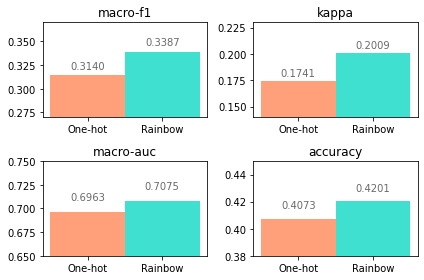Hyperparameters

The following plots show metric dynamics depending on every hyperparameter keeping other hyperparameters constant. These plots also clearly demonstrate the superiority of the Rainbow method for every hyperparameter and every metric.

Figure 3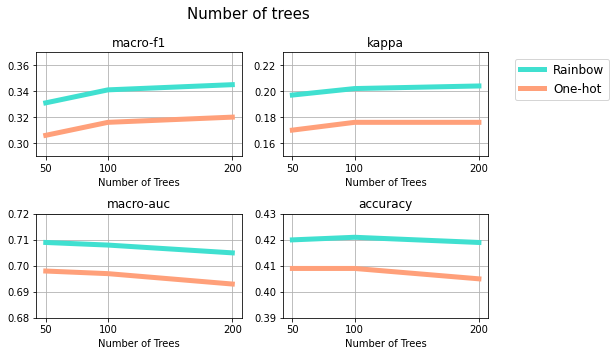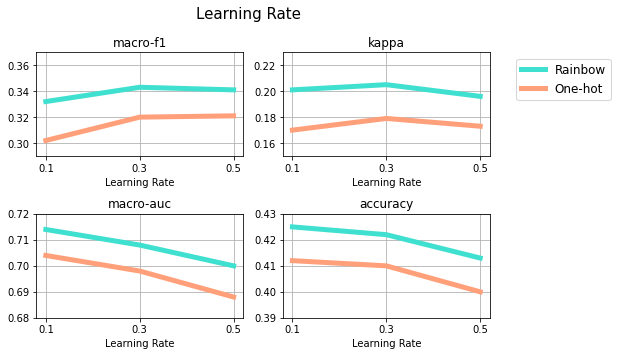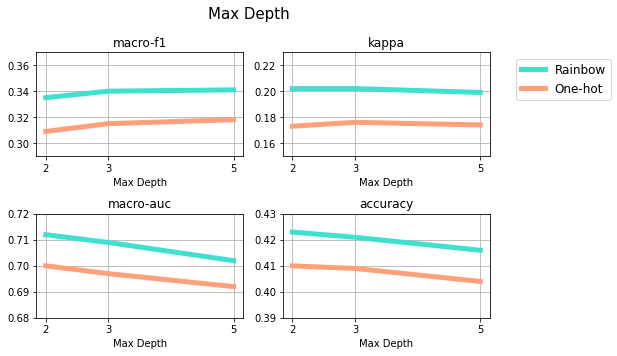Runtime

Next, let's compare the runtime for each method.

One-hot: 66.074 s
Rainbow:  7.444 s

The average time to run a single rainbow model is 9 times faster than that of the single One-hot model! So, in addition to a substantial increase in model performance metrics, the Rainbow method can also save data scientists a huge amount of time.

Interval, Ordinal, and Nominal

Next, we ran the models that covered the bundles of interval, ordinal, and nominal features separately. Below are the results.

Figure 5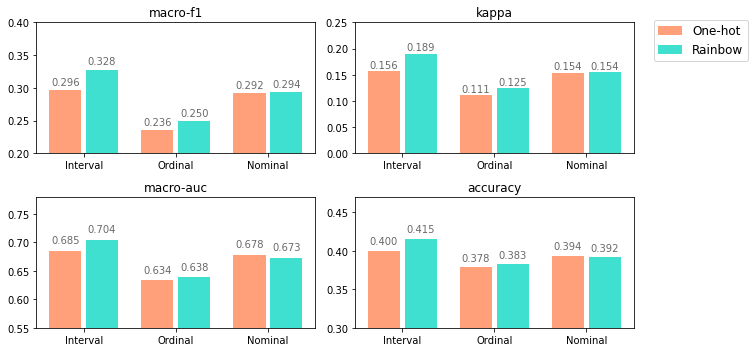These results demonstrate again that Rainbow is preferred to One-hot. As expected, interval and ordinal features gain the most from Rainbow encoding, while nominal variables - less so.

Feature Selection

Finally, to make a comparison fairer in terms of dimensionality, we picked top 10, top 50, and top 100 features from each feature set - Rainbow and One-hot. We used the feature importance attribute of the XGBoost model and aggregated feature importance scores for 4 cross-validation folds on the best hyperparameter set for each encoding type. Below are the results.

Figure 6Every single metric is considerably higher for the Rainbow method, especially with the lower number of selected features. As mentioned in Part I, rainbow encoding with 50 features is even better than One-hot with 100 features as shown by macro-f1, kappa, and accuracy. Also, note the drop from 50 to 10 features in One-hot versus rainbow - macro-f1 loses 0.065 points with One-hot compared to only 0.016 points with Rainbow.

Conclusion

As we illustrated above, the Rainbow method is an elegant and simple way to encode categorical variables, that will significantly reduce data dimensionality without losing any part of valuable signal; that will likely cause substantial improvements in model performance metrics (or, at the very least, will not cause any reductions in metrics); and that will save a great amount of time for modelers.

In Part III we provide mathematical justification of the Rainbow method and demonstrate its superiority against One-hot.

The Rainbow Method Part III. Mathematical Foundation.

In this article, we justify mathematically the advantages of the Rainbow method over One-hot. We start with a simple example, and then we proceed with a full generalization and a simulation. In the final part, we discuss various assumptions and limitations of the Rainbow method and provide actionable recommendations to get the best out of Rainbow. Please note that the discussion below applies to any tree-based algorithm that relies only on the order of the values. That includes any random forest and any gradient boosting algorithm such as xgboost.

How exactly does rainbow help?

Simple Example

Consider the following example:

• assume a binary classification problem, with output variable having values 0 and 1;
• assume we have only one categorical variable V and no other variables;
• assume V has exactly four categories with values v1 < v2 < v3 < v4;
In the case of the nominal variable, the values simply represent numeric codes assigned to each category.
• assume each value of V has the same or close number of samples in the data;
• assume an ideal separation will give to {v1 & v2} samples f-score = 1 and to {v3 & v4} samples f-score = 2, where f-score represents some decision function used for separation (e.g. Gini impurity, information gain, etc.).
I.e. the data generating process would separate between the groups {v1 & v2} and {v3 & v4}, but would not distinguish values within each group.

Let's consider two cases - Rainbow and One-hot. For Rainbow, we will use V directly to build a decision tree. For One-hot, we will need to create four binary variables - let's call them V1, V2, V3, V4, where Vi = 1 if V=vi and 0 otherwise.

Let's for simplicity build a single decision tree. Assume a simple algorithm that picks each next feature randomly, and compares all values of that feature to make the best split. In the case of the rainbow, samples from two categories will be sent to the left branch and samples from two other categories will be sent to the right branch of the tree. In such a situation a natural split occurs between v2 and v3, and rainbow would need one split,

Figure 1

while One-hot would need two or three splits. Here are two examples:

Figure 2

Figure 3

In other words, assuming a clear order between categories, the Rainbow method is strictly better at separating samples - it makes fewer splits. In this example, it finds the single perfect split between v2 and v3. The One-hot method goes through four scales instead of one and does not naturally incorporate a combination of information from a few features.

Figure 4

But what happens if the categories do not go in perfect order?

Generalizing simple example

Let's look at the more general case, where the values of the categorical variable are in a different order. Below are some examples of 4 categories and their f-scores. The same f-score justifies combining values into a group. For instance, 1,1,2,2 represents our example above, where the first two categories get assigned f = 1, and the last two categories get assigned f = 2, and the split should happen in the middle.

The number of splits would depend on the order in which these categories appear.

For rainbow orders:

• 1,1,2,2 and 2,2,1,1 the number of splits would be 1;

Figure 5

• 1,2,2,1 and 2,1,1,2 the required number of splits would be 2;

Figure 6

• 1,2,1,2 and 2,1,2,1, the required number of splits would be 3.

Figure 7

For One-hot, the orders below would represent the order in which the One-hot features are selected and added to the tree:

• 1,1,2,2 and 2,2,1,1 the required number of splits would be 2;

As in our simple example above (Figure 2), the first split would be made on V1, the second on V2, and that would be sufficient to separate between the samples with f = 1 and f = 2.

• 1,2,2,1 and 2,1,1,2 and 1,2,1,2 and 2,1,2,1 the number of splits would be 3.

In these examples, as shown above (Figure 3), three splits would be needed given the order in which binary features enter the tree.

Note that the Rainbow and One-hot orders described here are not the same orders, so we cannot compare them directly. In the case of Rainbow, we look at the order at the top - in which categories appear on a single scale, while for One-hot we look at the order at the bottom - in which categories are picked by the model. Nevertheless, we see that on average Rainbow is better as it provides a lower number of splits - best case 1, average case 2, worst case 3; while One-hot gives best case 2, average case $\frac 83$, and worst case 3. In fact, this is true in general and we show it below.

General Case: Random rainbow vs One-hot

Assume that a certain categorical variable $V$ has $K$ categories with values v1 < v2 < v3 ... < vk. Then:

• One-hot would create $K$ features $V1, V2, ..., VK$, where $Vi$ = 1 if $V$ = vi and 0 otherwise;
• Rainbow would create a single feature, where all the categories appear on one scale. We can keep the name of this variable $V$.

Assume $K$ categories optimally would need to be split into $g$ groups of size $G_1,G_2,...,G_g$. By size $G_i$ we mean a number of categories out of $K$ (not the number of samples).

For example, in the simple example above - the order is 1,1,2,2:

Figure 8

and $K=4$, $g=2$, $G_1=2$ consisted of {v1, v2}, and $G_2=2$ consisted of {v3, v4}.

Another example - if the order is 1,2,2,1:

Figure 9

Here $K=4$, $g=2$, $G_1=2$ consists of {v1, v4}, and $G_2=2$ consists of {v2, v3}.

Unlike the simple example of the perfect rainbow, in the general case, the $K$ categories would appear in random order, and our rainbow would be random. However, for the purposes of computation, we only care about the groups of the same f-values rather than about actual categories. In other words, if v1 and v2 samples produce the same f-score, it does not matter whether the order is (v1, v2) or (v2, v1).

For One-hot the order that would matter is the one in which One-hot splits are made. This order is also assumed to be random. This is the order that we would always refer to when talking about One-hot.

In reality, the One-hot splits are not fully random and the order of them being picked by the model varies depending on the number of samples in each category, subsamples of columns chosen, and other hyperparameters. That can be both in favor and against the case for One-Hot. We will come back to this assumption later in the article (see Randomness Assumption), and for now, will proceed with assuming randomness for simplicity.

While the orders that matter for Rainbow and One-hot are not the same, it helps to couple them to make comparisons. Let's compute the required number of splits in best, average, and worst cases.

Main Comparison Metric

As in the simple example above, in this general case of random rainbow vs One-hot, we will compare the number of splits made by the model to approximate the data generating process. Why is it our main metric? Less splits means:

• less time;
• less computational resources;
• simpler model;
• less overfit;
• more effective model.

Best case

One-hot

In the best-case scenario for One-hot, the biggest group won't be split.

For example, in the case of 1,1,2,2,2,3,3 we would like the biggest group 2,2,2 to end up on the bottom of the tree, and thus, not introduce any extra splits.

Figure 10

Thus, in the best case, One-hot would require $K - G_{max}$ splits, where $G_{max} = max_i G_i$ is the maximum of $G_i$. This best-case $K - G_{max}$ is obtained when each group except the largest one gets one split per category and the remaining largest group has the same answer so doesn't require any additional splits. To prove that one cannot do better, observe that if a few categories do not appear on any tree node, i.e. no splits are made, then they are "combined" - there is no way to distinguish the values in these two categories.

Rainbow

In the best case of a rainbow - the values are in perfect order, and the minimum splits are needed. Given $g$ groups, that would require $g-1$ splits. This happens when groups are together and it simply splits at $g-1$ points to separate $g$ groups.

For example, clearly, two splits are needed for the perfect rainbow with three groups 1,1,2,2,2,3,3.

One cannot do better since if there is no split between two groups there is no way to distinguish them.

Comparison

Clearly $$K - G_{max}=(\sum_{i=1}^g G_i)-G_{max}=(\sum_{i!=max}G_i) \geq (\sum_{i!=max} 1) = g-1$$ and hence best rainbow is better than the best One-hot.

Average Case

One-hot

From the logic presented in the best case, it follows that

The number of splits that One-hot needs would be $N$ - the number of categories that are chosen before the remaining ones are in one group.

Figure 10 shows the best case - where all the remaining ones belong to the largest group. In the average case, the remaining group can be any group or any group's part. We cannot expect that a homogenous group won't be broken into pieces when building an average tree. What we care about is that the tree building should end when reaching some homogenous group.

In the best case $N = K - G_{max}$. In the average case $N = K - R$ where $R$ is the size of the remaining group.

For example, if the order in which One-hot categories are chosen for the tree is 1,1,2,2,3,3,2,2,2 then $R=3$ since the last group 2,2,2 is of size 3. The number of categories $K=9$, thus the number of splits would be $K-R=6$.

It is easiest to compute the expected value of $N$ by using less common formula $\mathbb E[X] = \sum_{j} \mathbb P[X \geq j]$ applied to $R$. We then utilize the formula of hypergeometric distribution to calculate each probability and achieve the result below.

\begin{align} \mathbb E[N] &= \mathbb E[K-R] = K- \mathbb E[R] = K - \sum_{j=1}^{K} \mathbb P[R \geq j] =K- \sum_{j=1}^K \frac{\sum_i \frac{G_i!}{(G_i-j)!}} {\frac{K!}{(K-j)!}} = (K-1) - \sum_{j=2}^K \frac{\sum_i \frac{G_i!}{(G_i-j)!}} {\frac{K!}{(K-j)!}}\\ \end{align}

Rainbow

From the best case logic, it also follows that

The number of splits that random Rainbow needs would always be $D$ - the number of consecutive pairs in rainbow order that are in distinct groups.

In the best case $D=g-1$.

For example, in the case of Rainbow order 1,1,2,2,3,3,2,2,2 the splits will happen in 3 places, and these are the only places where the group is changed and the values in consecutive pairs belong to distinct groups.

Let's calculate the expected value of $D$. We do this by calculating for $K-1$ consecutive pairs as potential places for splitting the probability that the f-score is different. It is easier to subtract from one the probabilities that f values in each consecutive pair are the same. We sum through all possible groups $i$ to compute the likelihood that two consecutive values belong to one group.

\begin{align} \mathbb E[D] &= (K - 1) \cdot (1-\frac{ \sum_i G_i \cdot (G_i-1)} {K(K-1)}) \notag \\ &= (K-1)-\frac{ \sum G_i \cdot (G_i-1)} {K} \notag \end{align}

Comparison

An easy way to see that $\mathbb E[D]$ is larger than $\mathbb E[N]$ is the stochastic coupling since both $N$ and $D$ can be obtained as starting from $K-1$ and subtracting $1$ for each consecutive pair in the same group; except in case of $N$, all these pairs have to be in the string at the end. Due to this restriction $N$ is always larger.

Worst Case

One-hot

In the worst case for One-hot, the number of splits is $K-1$ when one goes through all but one category to get to one group remaining.

Rainbow

In this case, the number of needed splits is

$\min( (K - 1), 2 \cdot (K-G_{max}))$

as we can only get up to two splits per each category that is not in the largest group.

Indeed, if there are no consecutive pairs of values that belong to the same group then the number of splits would be $K-1$, for example here 3,2,1,3,1,2.

At the same time, if there is a prevalent group and some minority groups, for example, 1,1,1,2,1,1,3,1,1, the number of splits will equal to the number of minority groups - $K-G_{max}=2$ in this case (2 and 3) times 2 which is the maximum number of splits that each minority group introduces - one to the left and one to the right.

Thus the needed number of splits will be the minimum between the two cases above.

Comparison

Clearly, the number of splits for Rainbow is less than or equal to that of One-hot for the worst case as well.

Simulation

We have shown above that theoretically in a general case Rainbow method outperforms One-hot in best, average, and worst-case scenarios. Let's look at the empirical simulation: we applied the formulas for best, average, and worst-case number of splits for Rainbow and One-hot to compare the distribution of splits for some examples of the data generating processes.

Figure 11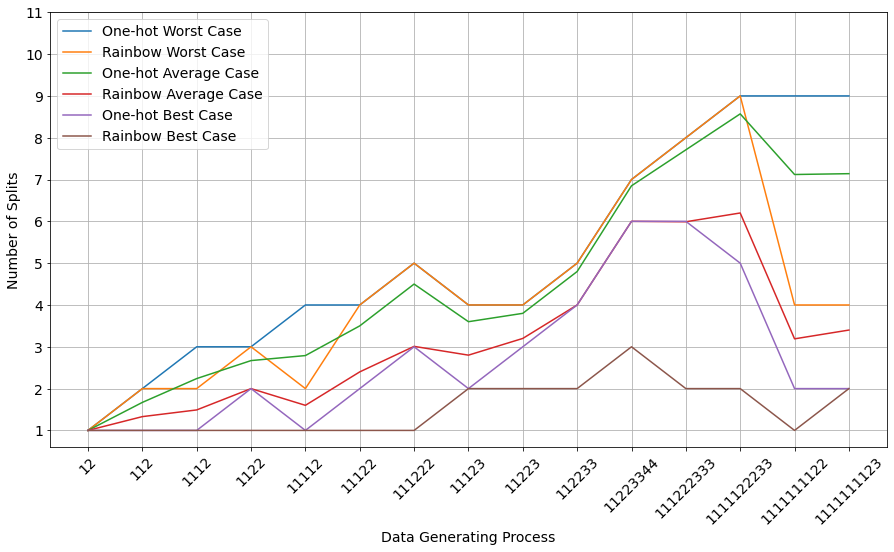One can clearly see that the simulation confirms our theoretical finding:

• Best Rainbow always needs a less or equal number of splits than Best One-hot: the brown line is almost always below the purple line;
• Average Rainbow always outperforms Average One-hot: the red line is always strictly below the green line;
• Worst Rainbow needs the same or a lower number of splits than worst One-hot: the orange line is either below or coincides with the blue line.

Conclusion

Discussion of Assumptions

While the results above appear to be very optimistic, we should discuss the method limitations and assumptions we made.

Single Tree Assumption:The use of a single decision tree vs forest.

That assumption does not really impact the outcomes, since a single tree is equivalent to a forest of trees with depth 1. In fact, if our method works on simpler models that only adds to its advantages.

Randomness Assumption:One-hot picks columns randomly.

We assumed above that our machine learning algorithms pick features somewhat randomly, and then make a split by using full scales of values of those features. One might argue that in reality, before making any split, algorithms such as xgboost would look at many splits across all features and will pick the best.

We understand that this is a strong assumption and in reality, the One-hot splits are not fully random. However, they are also not fully deterministic. First, most of the algorithms don't run all possible comparisons within all features to make a choice about the node, rather some quick set of rough comparisons, which is done to avoid overfitting. Second, various hyperparameters have a substantial effect on which split will be chosen: subsample of columns, subsample of rows, maximum depth, etc. Third, if we are talking about bigger categorical variables with many categories - One-hot creates a lot of features, and randomness assumption would be not far from the truth. Finally, counts of samples for each category can be highly uneven, and that also gets us closer to randomness and is likely to yield worse results for One-hot than for Rainbow.

How to find the best Rainbow?

As we have shown above the distribution of the number of splits is strictly better for Rainbow vs One-hot. However, one might argue that in reality we might be choosing between a good One-hot (assuming intelligent algorithms and relaxing randomness assumption) and a random rainbow.
In fact, the quality of a rainbow is an unknown. We can see that the Average Rainbow (red line) is between Best One-hot (purple line) and Average One-hot (green line). So it is important to try and find a good order rainbow, that approaches the data generating process.

The proposed solution here is very simple and clear - pick a few, choose the best. We invite you to try a few possible Rainbow encodings and compare them side by side to each other and One-hot. Your data and problem context will be the best tools here to choose the best encoding. We also recommend you to look at a few metrics, as well as model runtime. We expect that the Rainbow method would provide you with much better overall results, especially if you have many categorical variables with many values.

Final Notes

This article just opens the conversation about the Rainbow method, and by no means exhausts the topic. In potential future investigations, we could explore some other aspects. To name a few: continuous target variables; comparison with other dimensionality reduction methods, such as PCA; missing values and how they fit the Rainbow framework; limitations and implications of combining seemingly unrelated features into a single Rainbow. We hope to open the gate for further questions and feedback on this method.

While the topic of One-hot and binary-One-hot encoding has been touched by many authors:

the approach presented here is rarely used in industry or academia, while has shown superior results and by definition creates fewer features.